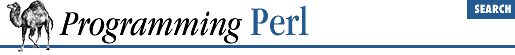home | O'Reilly's CD bookshelfs | FreeBSD | Linux | Cisco | Cisco Exam3.2.188 vec

vec

EXPR

,

OFFSET

,

BITS

This function treats a string (the value of EXPR ) as a vector of unsigned integers, and returns the value of the element specified by OFFSET and BITS . The function may also be assigned to, which causes the element to be modified. The purpose of the function is to provide very compact storage of lists of small integers. The integers may be very small - vectors can hold numbers that are as small as one bit, resulting in a bitstring.

The OFFSET specifies how many elements to skip over to find the one you want. BITS is the number of bits per element in the vector, so each element can contain an unsigned integer in the range 0..(2** BITS ) -1 . BITS must be one of 1 , 2 , 4 , 8 , 16 , or 32 . As many elements as possible are packed into each byte, and the ordering is such that vec(\$vectorstring,0,1) is guaranteed to go into the lowest bit of the first byte of the string. To find out the position of the byte in which an element is going to be put, you have to multiply the OFFSET by the number of elements per byte. When BITS is 1, there are eight elements per byte. When BITS is 2, there are four elements per byte. When BITS is 4, there are two elements (called nybbles) per byte. And so on.

Regardless of whether your machine is big-endian or little-endian, vec(\$foo, 0, 8) always refers to the first byte of string \$foo . See select for examples of bitmaps generated with vec .

Vectors created with vec can also be manipulated with the logical operators | , & , ^ , and ~ , which will assume a bit vector operation is desired when the operands are strings.

A bit vector ( BITS == 1 ) can be translated to or from a string of 1s and 0s by supplying a b* template to unpack or pack . Similarly, a vector of nybbles ( BITS == 4 ) can be translated with an h* template.